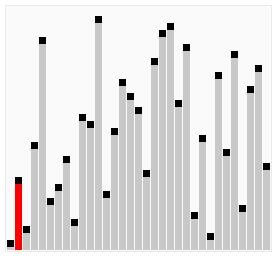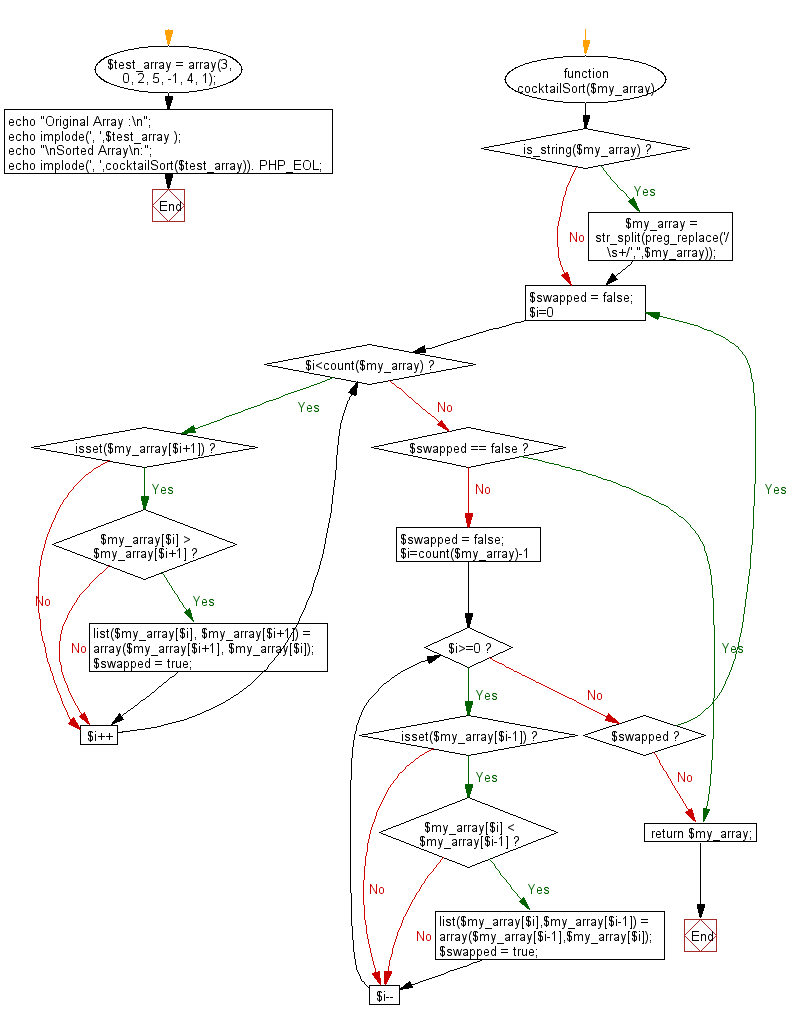﻿ PHP: Sort a list of elements using Cocktail sort - w3resource

# PHP Searching and Sorting Algorithm: Cocktail sort

## PHP Searching and Sorting Algorithm: Exercise-7 with Solution

Write a PHP program to sort a list of elements using Cocktail sort.

Cocktail shaker sort (also known as bidirectional bubble sort, cocktail sort, shaker sort, ripple sort, shuffle sort, or shuttle sort ) is a variation of bubble sort that is both a stable sorting algorithm and a comparison sort. The algorithm differs from a bubble sort in that it sorts in both directions on each pass through the list. This sorting algorithm is only marginally more difficult to implement than a bubble sort and solves the problem of turtles in bubble sorts. It provides only marginal performance improvements, and does not improve asymptotic performance; like the bubble sort, it is not of practical interest, though it finds some use in education.

Visualization of shaker sort :Sample Solution :

PHP Code :

``````<?php
function cocktailSort(\$my_array)
{
if (is_string(\$my_array))
\$my_array = str_split(preg_replace('/\s+/','',\$my_array));

do{
\$swapped = false;
for(\$i=0;\$i<count(\$my_array);\$i++){
if(isset(\$my_array[\$i+1])){
if(\$my_array[\$i] > \$my_array[\$i+1]){
list(\$my_array[\$i], \$my_array[\$i+1]) = array(\$my_array[\$i+1], \$my_array[\$i]);
\$swapped = true;
}
}
}

if (\$swapped == false) break;

\$swapped = false;
for(\$i=count(\$my_array)-1;\$i>=0;\$i--){
if(isset(\$my_array[\$i-1])){
if(\$my_array[\$i] < \$my_array[\$i-1]) {
list(\$my_array[\$i],\$my_array[\$i-1]) = array(\$my_array[\$i-1],\$my_array[\$i]);
\$swapped = true;
}
}
}
}while(\$swapped);

return \$my_array;
}
\$test_array = array(3, 0, 2, 5, -1, 4, 1);
echo "Original Array :\n";
echo implode(', ',\$test_array );
echo "\nSorted Array\n:";
echo implode(', ',cocktailSort(\$test_array)). PHP_EOL;
?>
```
```

Sample Output:

```3, 0, 2, 5, -1, 4, 1
Sorted Array :
-1, 0, 1, 2, 3, 4, 5
```

Flowchart :PHP Code Editor:

Have another way to solve this solution? Contribute your code (and comments) through Disqus.

What is the difficulty level of this exercise?

Test your Programming skills with w3resource's quiz.

﻿